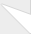# Introduction to C++ Templates

This tutorial will give you a quick introduction to C++ templates. You will not be a template guru after reading it but you will be able to understand a lot of the "templated" code that's out there.

toc_collapse=0;
Contents

### Motivation

What problems do templates solve or, even better, why should you use them? Templates allow you to parameterize types. You can use them to create the so called "generic" classes and "generic" functions. That means you can generalize the type of

• Class members
• Function parameters and return type

### Proof of concept: Function templates

Here's one scenario where you would find templates very useful. How often do you find yourself swapping values in your code? You probably have a swap function written like this...

//      A classic implementation of a swap function
void swap(int& first, int& second)
{
int temp = first;
first = second;
second = temp;
}

Now comes the key question. What if you want to swap double's instead of int's? What if you want to swap char's or a structure data type? Are you gonna rewrite the same code again, knowing the only thing you are going to change is the type of the two parameters? Well... you will end up doing that if you don't know about templates.

With templates, you can add a template parameter that specifies the type of the data your swap function is going to swap. The syntax for this might seem awkward at first but don't worry as you will soon get used to it.

template<class T>
void swap(T& first, T& second)
{
T temp = first;
first = second;
second = temp;
}

Congratulations! This is your first function template. Your new swap function can now swap variables of any type (as long as that type is assignable of course, i.e. it defines an = operator). You can call swap with any variables of pretty much any type.

int integerOne = 1, integerTwo = 2;
swap(integerOne, integerTwo);

double pi = 3.14159265358979, e = 2.7182818284590;
swap(pi, e);

//      Let "Object" be your own custom defined data type...
Object firstObject, secondObject;
swap(firstObject, secondObject);

### Proof of concept: Class templates

You can apply the same concept to classes. Consider the following. How often have you found yourself writing a container class for one of your projects? Let's say you were writing a stack class. It would be nice if your stack class was able to store any type of variables as opposed to simply storing numbers or void pointers. Templates can once again help you.

This is how you would write your stack class that only stores integers...

class Stack
{
public:
Stack() : _storage(new int), _size(0) {}
~Stack() { delete [] _storage; }
Stack(const Stack& copy);

public:
void pop();
int top() const;
void push(int data);
bool empty() const;
unsigned int size();

private:
int * _storage;
unsigned int _size;
};

But what if you want to store an std::string or some other custom-defined data type in your stack. Oops, you can't even use casting to get around that! Let's add a template parameter to our Stack class to generalize the type of the elements our stack stores and thus solve our problem.

//      We are now going to write the Stack class
//      in terms of "T" as opposed to "int"
template<class T>
class Stack
{
public:
Stack() : _storage(new T), _size(0) {}
~Stack() { delete [] _storage; }
Stack(const Stack<T>& copy);

public:
void pop();
T top() const;
void push(const T& data);
//      You could define methods inline
bool empty() const { return _size == 0; }
unsigned int size() { return _size; }

private:
T * _storage;
unsigned int _size;

};

//      This is the syntax for defining a non-inline method of a class template...
void Stack<T>::pop()
{
//      TODO: Check if the stack is empty
_size--;
}

T Stack<T>::top() const
{
//      TODO: Check if the stack is empty
return _storage[_size-1];
}
void Stack<T>::push(const T& data)
{
//      TODO: Check if there's room first.
_storage[_size++] = data;
}

You might have noticed how I changed the pass by value mechanism to pass by const reference in void Stack::push(const T&). Generally, you will find passing by const reference to be a very wise choice since the objects you will end up storing in your generic stack will probably be bigger than primitive integers and thus passing them by value would be very expensive.

This is how you would instantiate a stack object that stores std::strings.

#include <string>

//      Notice the new syntax.
//      You are specifying the type by
//      writing it between the angle brackets.
Stack<std::string> stringStack;

//      You can also have a stack of ints...
Stack<int> intStack;

//      Or a stack of char *
Stack<char *> cstringStack;

### A small warning

As a final note on class templates you should know that class and function templates force you to have their declaration and their definition in the same file. This means that you can't declare a function template in a .h file and then implement it in a .cpp file. You are going to have to write all of it in the .h file. Same thing for class templates. You cannot implement the class methods in a different .cpp file, it has to be in the same .h file. This restriction has to do with the way compilers generate the code for templates, and yes, it will get very annoying.

### Great job on this tutorialGreat job on this tutorial Alin.